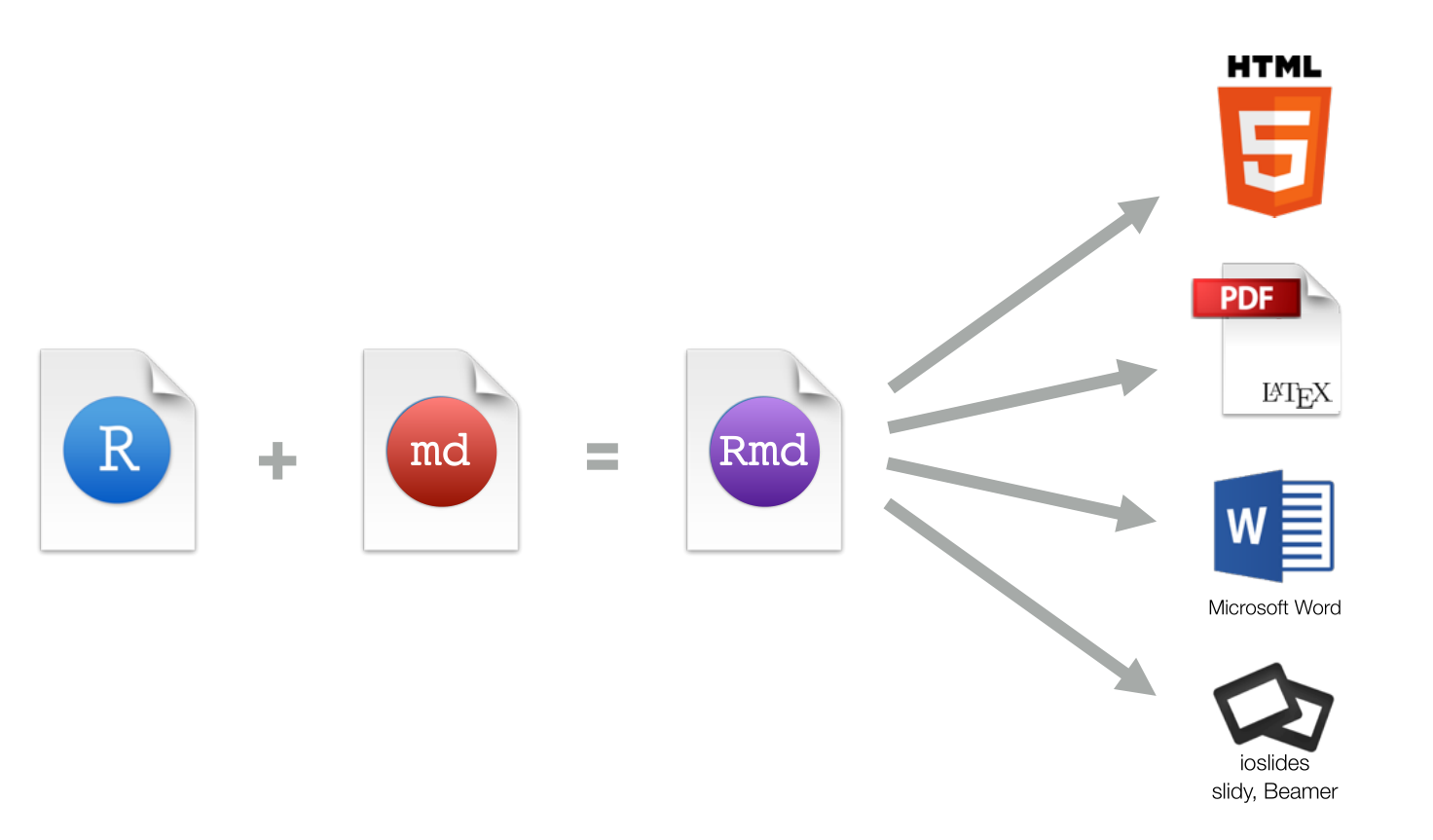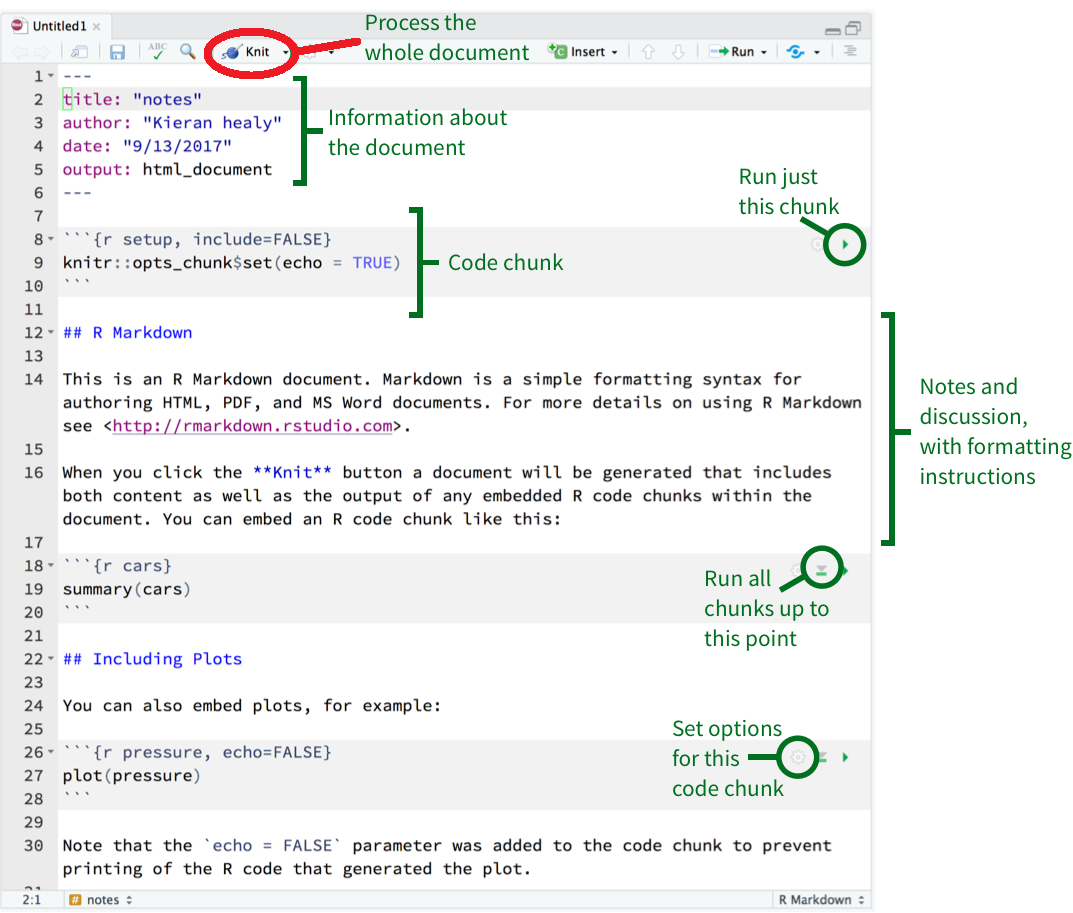# 第 4 章 可重复性报告

## 4.1 什么是Rmarkdown## 4.2 markdown 基本语法

# This is a title

#  第一章   （注意   "#" 与 "第一章"之间有空格）
##  第一节  （同上，"##" 与 "第一节"之间有空格）

This is a sentence.

Now a list begins:

- no importance
- again
- repeat

A numbered list:

1. first
2. second

__bold__, _italic_, ~~strike through~~

## 4.3 Hello R Markdown

Rstudio create Rmd file ： File -> New File -> R Markdown.

• text
• code## 4.4 生成html文档

---
title: Habits
author: John Doe
date: "2020-09-17"
output:
html_document:
df_print: paged
toc: yes
number_sections: yes
---

## 4.5 生成word文档

rmarkdown 生成的word功能不是很多，推荐使用officedown , officer, flextable宏包

## 4.6 生成pdf文档

pdf文档可以插入漂亮的矢量图和优雅的数学公式，所以备受同学们的喜欢。但往往我们写中文的时候，编译不成功。这里就来讲讲如何解决这些问题，推荐阅读这里，或者看这个视频

Instructions

1. 安装最新版本 R (>3.5) 和 RStudio Desktop (>1.2).
2. 安装pdf查看器，sumatrapdf网站
3. 安装 LaTeX. 然而这个软件会比较大 (e.g. MacTeX is approximate 3.9G). 如果你之前没有安装过 LaTeX，我推荐你安装轻量级的 tinytex. 安装方法如下，打开R，然后再命令行输入:
install.packages("tinytex")
tinytex::install_tinytex(dir = "D:\\Tinytex", force = T)

1. 修改头文件，用下面的内容替换Rmarkdown的头文件, 不要修改缩进
---
title: 'Going deeper with dplyr'
author: "王小二"
date: "r Sys.Date()"
output:
pdf_document:
latex_engine: xelatex
extra_dependencies:
ctex: UTF8
number_sections: yes
df_print: kable
toc: yes
classoptions: "hyperref, 12pt, a4paper"
---

## 4.7 使用方法

### 4.7.1 插入公式

$\frac{\sum (\bar{x} - x_i)^2}{n-1}$

$$\Theta = \begin{pmatrix}\alpha & \beta\\ \gamma & \delta \end{pmatrix}$$

$\Theta = \begin{pmatrix}\alpha & \beta\\ \gamma & \delta \end{pmatrix}$

### 4.7.2 插入图片

{r, out.width='35%', fig.align='left', fig.cap='this is caption'}
knitr::include_graphics("images/R_logo.png")### 4.7.3 运行代码

summary(cars)
##      speed           dist
##  Min.   : 4.0   Min.   :  2
##  1st Qu.:12.0   1st Qu.: 26
##  Median :15.0   Median : 36
##  Mean   :15.4   Mean   : 43
##  3rd Qu.:19.0   3rd Qu.: 56
##  Max.   :25.0   Max.   :120

### 4.7.4 表格

{r tables-mtcars}
knitr::kable(iris[1:5, ], caption = "A caption")


Sepal.Length Sepal.Width Petal.Length Petal.Width Species
5.1 3.5 1.4 0.2 setosa
4.9 3.0 1.4 0.2 setosa
4.7 3.2 1.3 0.2 setosa
4.6 3.1 1.5 0.2 setosa
5.0 3.6 1.4 0.2 setosa

### 4.7.5 生成图片

{r}
plot(pressure){r, out.width = '85%', fig.showtext = TRUE}
library(tidyverse)
library(nycflights13)
library(showtext)
showtext_auto()

flights %>%
group_by(dest) %>%
summarize(
count = n(),
dist = mean(distance, na.rm = TRUE),
delay = mean(arr_delay, na.rm = TRUE)
) %>%
dplyr::filter(delay > 0, count > 20, dest != "HNL") %>%
ggplot(mapping = aes(x = dist, y = delay)) +
geom_point(aes(size = count), alpha = 1 / 3) +
geom_smooth(se = FALSE) +
ggtitle("这是我的标题")